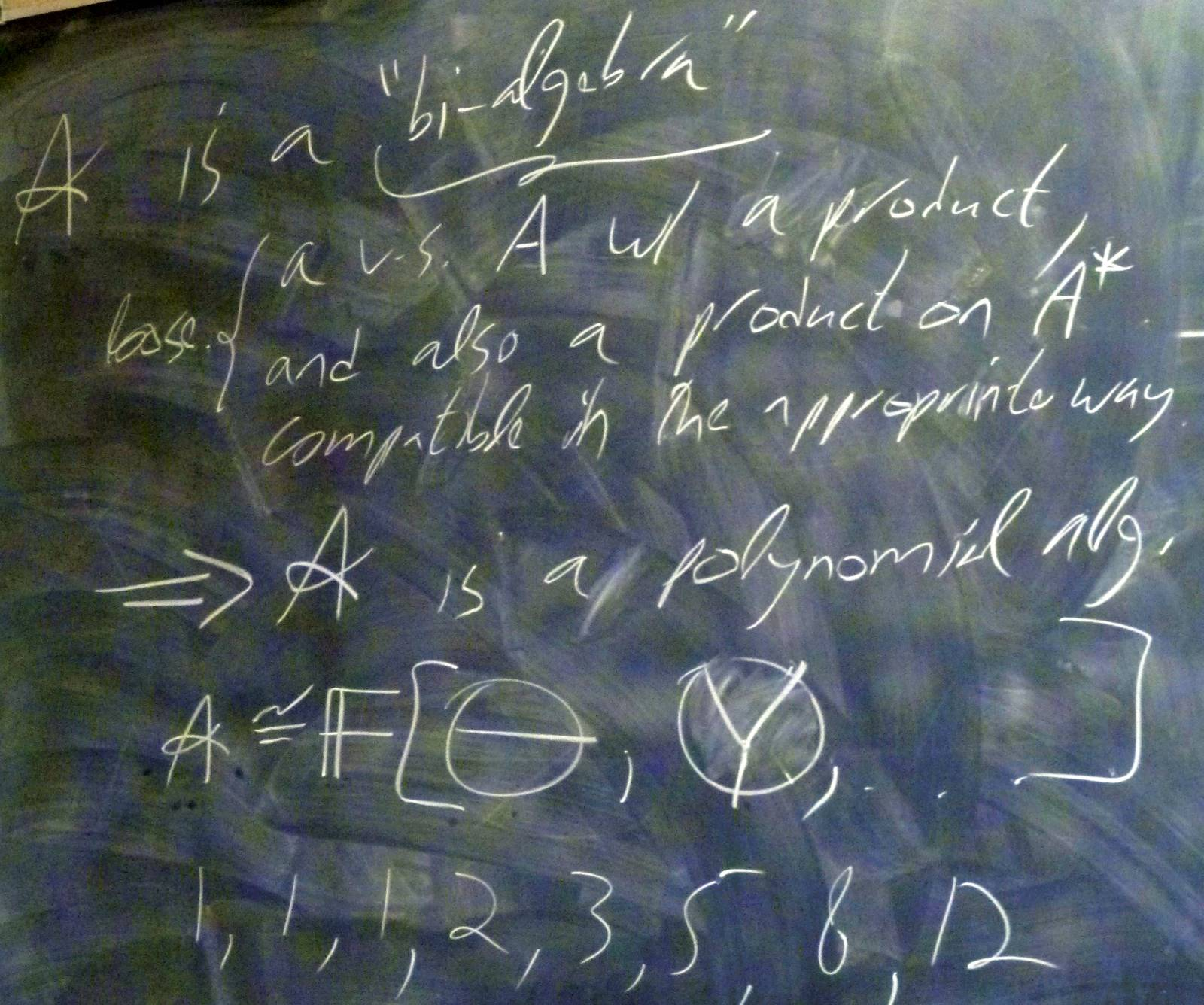© | Dror Bar-Natan: Classes: 2013-14: AKT: < >

# AKT-140310 Video

 width: 400 720 orig/AKT-140310.MOD For now, this video can only be viewed with web browsers that support

Notes on AKT-140310:    [edit, refresh]

The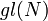$gl(N)$ weight system.

# Week of... Notes and Links
1 Jan 6 About This Class (PDF).
: Course introduction, knots and Reidemeister moves, knot colourings.
Tricolourability without Diagrams
: The Gauss linking number combinatorially and as an integral.
: The Schroedinger equation and path integrals.
Friday Introduction (the quantum pendulum)
2 Jan 13 Homework Assignment 1.
: The Kauffman bracket and the Jones polynomial.
: Self-linking using swaddling.
: Euler-Lagrange problems, Gaussian integration, volumes of spheres.
3 Jan 20 Homework Assignment 2.
: The definition of finite-type and some examples.
: The self-linking number and framings.
: Integrating a polynomial times a Gaussian.
Class Photo.
4 Jan 27 Homework Assignment 3.
: Chord diagrams and weight systems.
: Swaddling maps and framings, general configuration space integrals.
: Some analysis of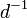$d^{-1}$.
5 Feb 3 Homework Assignment 4.
: 4T, the Fundamental Theorem and universal finite type invariants.
The Fulton-MacPherson Compactification (PDF).
: The Fulton-MacPherson Compactification, Part I.
: More on pushforwards,$d^{-1}$, and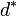$d^\ast$.
6 Feb 10 Homework Assignment 5.
: The bracket-rise theorem and the invariance principle.
: The Fulton-MacPherson Compactification, Part II.
: Gauge fixing, the beginning of Feynman diagrams.
R Feb 17 Reading Week.
7 Feb 24 : A review of Lie algebras.
: Graph cohomology and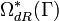$\Omega_{dR}^\ast(\Gamma)$.
: More on Feynman diagrams, beginning of gauge theory.
From Gaussian Integration to Feynman Diagrams (PDF).
8 Mar 3 Homework Assignment 6 (PDF)
: Lie algebraic weight systems.
: Graph cohomology and the construction of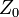$Z_0$.
Graph Cohomology and Configuration Space Integrals (PDF)
: Gauge invariance, Chern-Simons, holonomies.
Mar 9 is the last day to drop this class.
9 Mar 10 Homework Assignment 7 (PDF)
: The$gl(N)$ weight system.
: The universal property, hidden faces.
: Insolubility of the quintic, naive expectations for CS perturbation theory.
10 Mar 17 Homework Assignment 8 (PDF)
: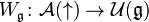$W_{\mathfrak g}\colon{\mathcal A}(\uparrow)\to{\mathcal U}({\mathfrak g})$ and PBW.
: The anomaly.
: Faddeev-Popov, part I.
Gaussian Integration, Determinants, Feynman Diagrams (PDF).
11 Mar 24 Homework Assignment 9 (PDF)
: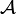${\mathcal A}$ is a bi-algebra.
: Understanding and fixing the anomaly.
Friday: class cancelled.
12 Mar 31 Monday, Wednesday: class cancelled.
: A Monday class: back to expansions.
E Apr 7 : A Friday class on what we mostly didn't have time to do.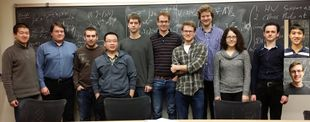Add your name / see who's in!
Dror's Notebookrefresh
panel
Managed by dbnvp: Click the "h:mm:ss" links on the right panel to jump to a specific video time.

0:16:39 [add] The $gl(N)$ structure constants.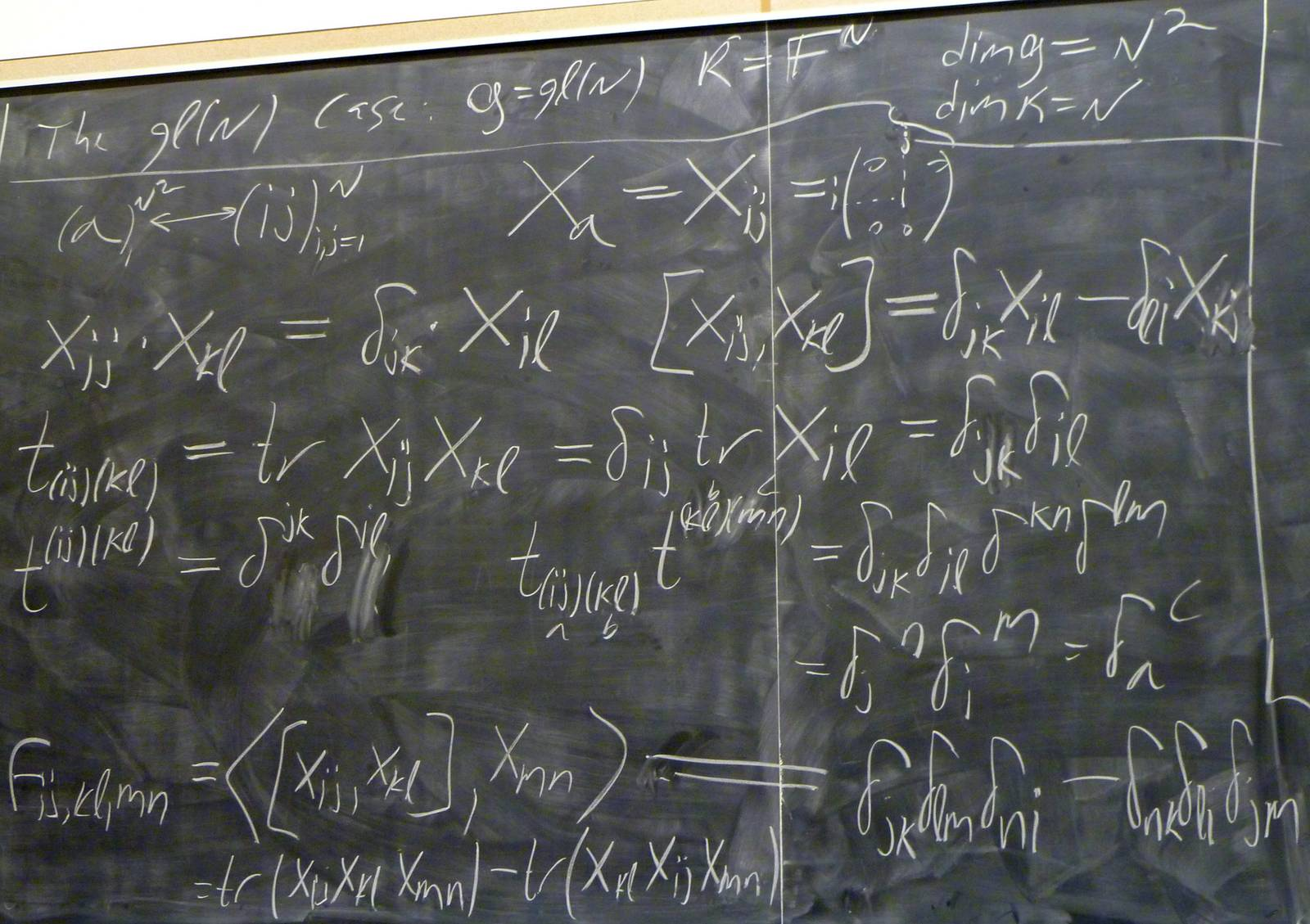0:27:25 [add] A $gl(N)$ computation.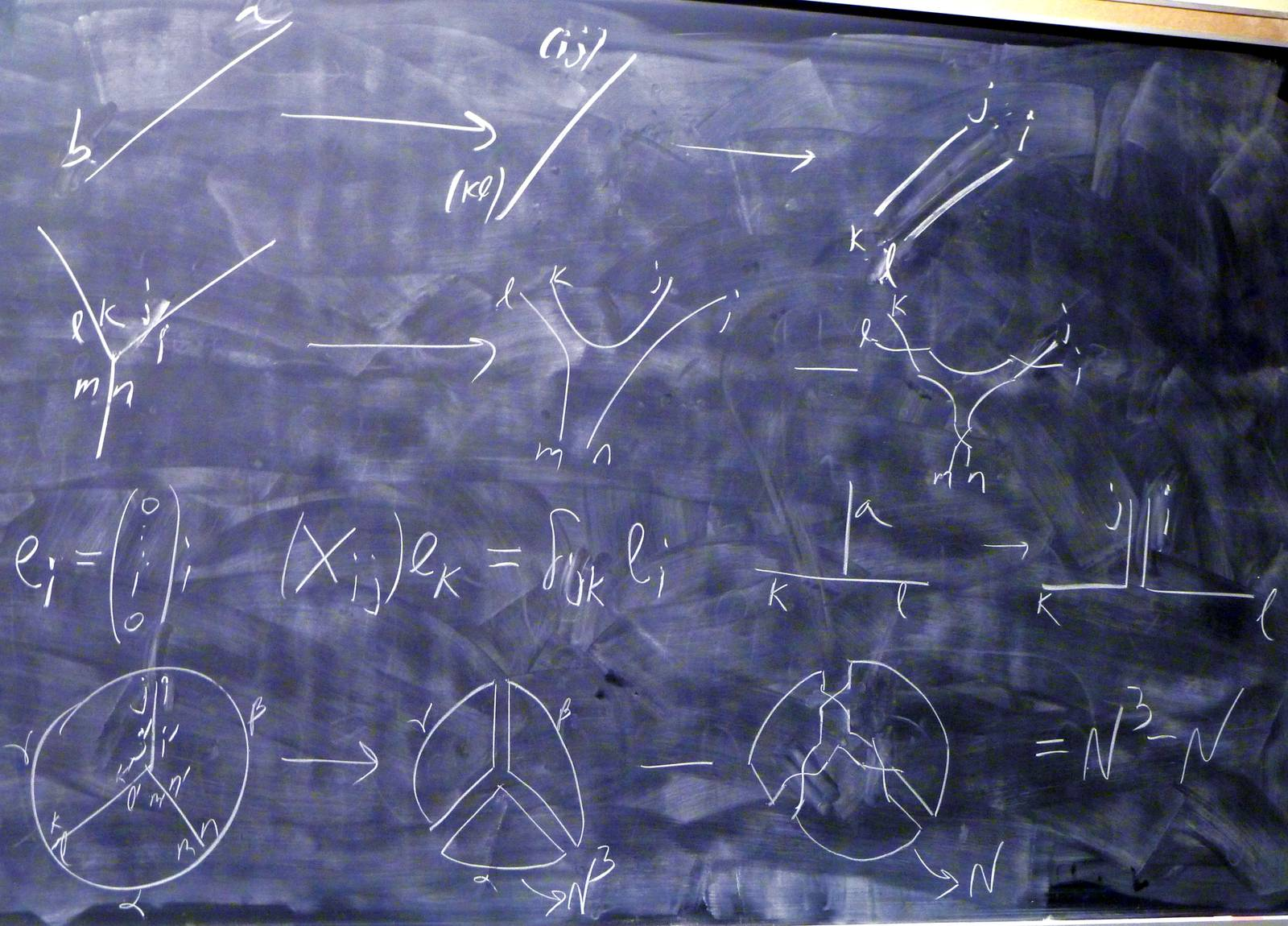0:31:53 [add] Further $gl(N)$ computations.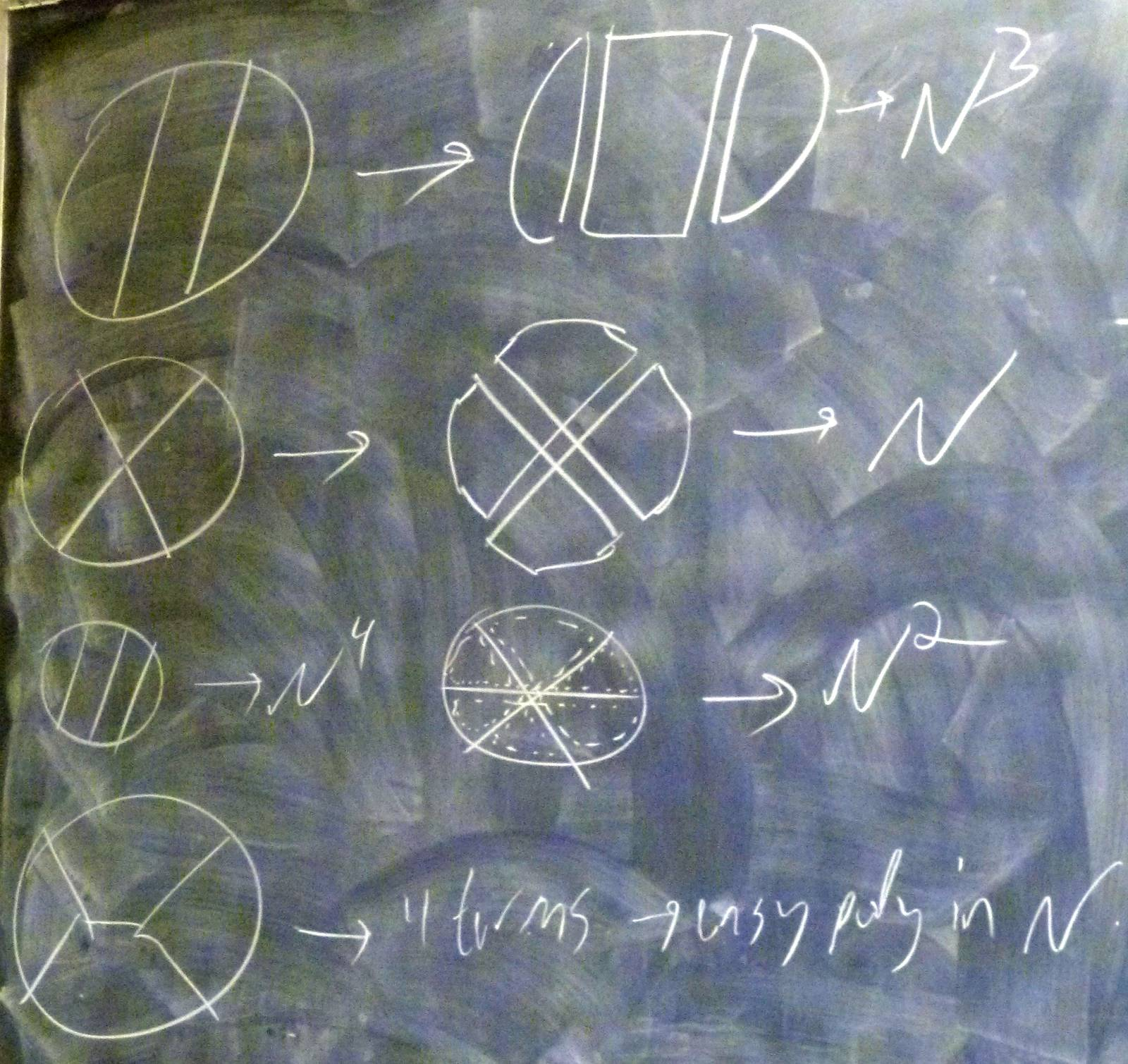0:35:43 [add] The $so(N)$ case.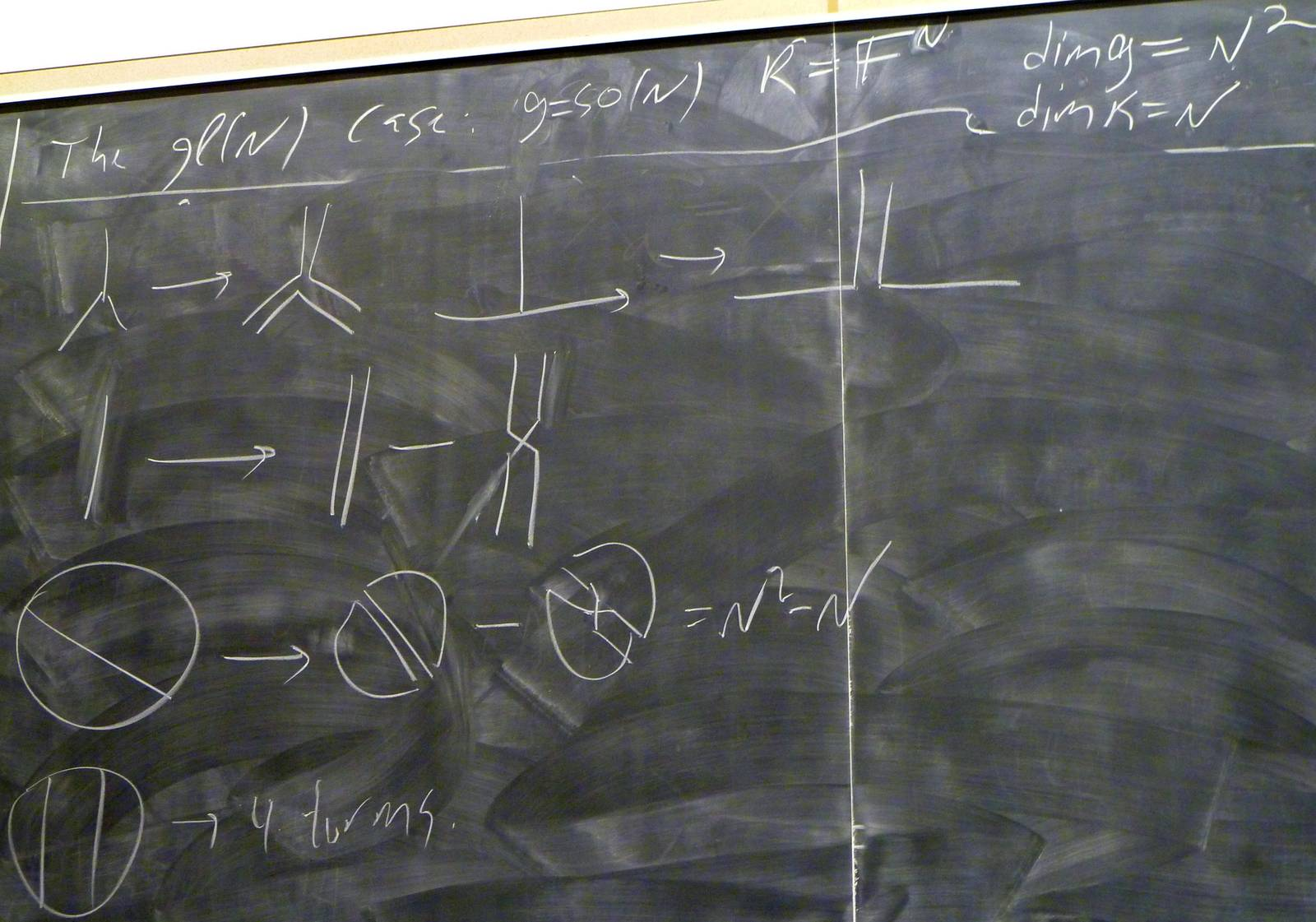0:35:45  In this note, we compute and interpret the structure constants $f_{abc}$ of $so(N)$, as well as the two-index tensors $t_{ab}$ encoding the information from the metric. In other words, we follow a similar process to the lecture, while disregarding the representation/skeleton edges.

Let $so(N) = \{Q \in gl(N) | Q^TQ = QQ^T = I, detQ = 1\}$, with the commutator as its bracket (i.e. $[A,B] = AB - BA$), and the metric $\langle A, B \rangle = tr(AB)$.

Let $\{\pm M_{ij}\}_{i < j}$ be a basis for $so(N)$, where $(M_{ij})_{kl} = \delta_{ij}\delta_{jl} - \delta_{il}\delta_{jk}$.

With this, compute $$t_{(ij)(kl)} = \langle M_{ij}, M_{kl} \rangle = tr(M_{ij}M_{kl}) = const\cdot\delta_{ik}\delta_{jl}$$

Note that this also gives us the inverses $t^{(ij)(kl)} = const\cdot\delta^{ik}\delta^{jl}$.

Now the structure constants: $$f_{(ij)(kl)(mn)} = \langle[M_{ij}, M_{kl}], M_{mn} \rangle = \langle M_{ij}M_{kl}, M_{mn} \rangle - \langle M_{kl}M_{ij}, M_{mn} \rangle$$ $$f_{(ij)(kl(mn)} = tr(M_{ij}M_{kl}M_{mn}) - tr(M_{kl}M_{ij}M_{mn}) = const\cdot\epsilon_{(ij)(kl)(mn)}$$

(With an appropriate choice of signs and ordering of the basis. In $so(3)$, an appropriate ordering and choice of signs is $\mathcal{B} = \{M_{12}, M_{23}, -M_{13}\}$.

If we order the basis, we can associate an integer lying somewhere from 1 to $N(N-1)/2$ (the dimension of $so(N)$) to each pair of indices $(ij)$, so the expression $\epsilon_{(ij)(kl)(mn)}$ makes sense - namely, let $a$, $b$, and $c$ correspond to $(ij)$, $(kl)$, and $(mn)$, respectively, and let $\epsilon_{(ij)(kl)(mn)} = \epsilon_{abc}$, the usual totally antisymmetric tensor.

Thus, up to a constant, $t^{(ij)(kl)} = \delta^{ik}\delta^{jl}$ and $f_{(ij)(kl)(mn)} = \epsilon_{(ij)(kl)(mn)}$.

As in the $gl(N)$ case, we can represent the result $t^{(ij)(kl)} = \delta^{ik}\delta^{jl}$ as a splitting of two lines in the diagram, as in the image below. In addition, we can represent the result $f_{(ij)(kl)(mn)} = \epsilon_{(ij)(kl)(mn)}$ as a trivalent vertex becoming a sum of diagrams, over transpositions of certain lines.

The diagram illustrated below goes to the following expression: $$I = \sum_{i,...,n,i',...,n'}f_{(ij)(kl)(mn)}t^{(ij)(i'j')}t^{(kl)(k'l')}t^{(mn)(m'n')}$$ $$I = \sum_{i,...,n,i',...,n'}\epsilon_{(ij)(kl)(mn)}\delta^{ii'}\delta^{jj'}\delta^{kk'}\delta^{ll'}\delta^{mm'}\delta^{nn'}$$ $$I = \sum_{i,...,n}\epsilon_{(ij)(kl)(mn)}$$

The last line exactly corresponds with the last illustration.

0:41:20 [add] $4T$ for $gl(N)$.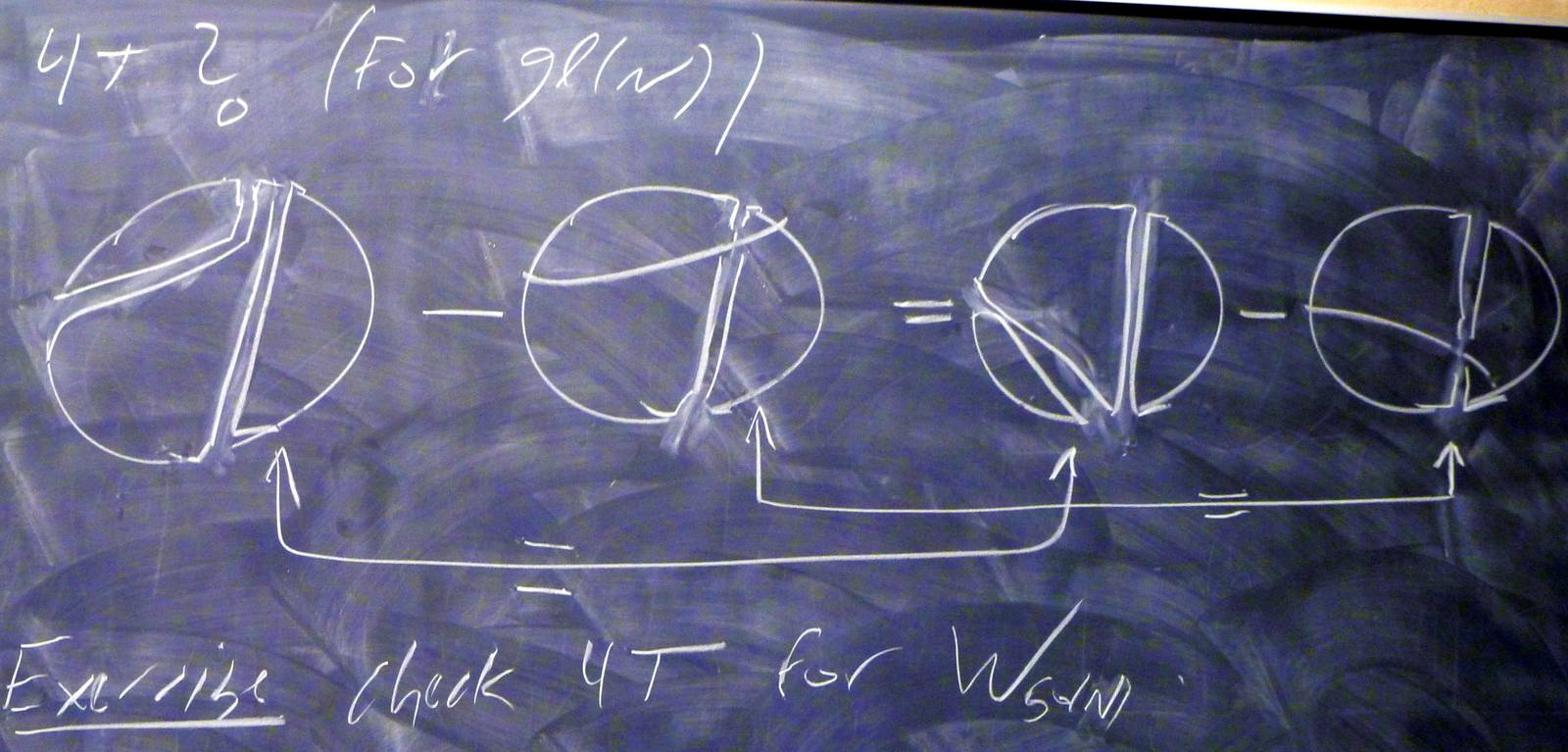0:48:21 [add] A preview of bi-algebras.